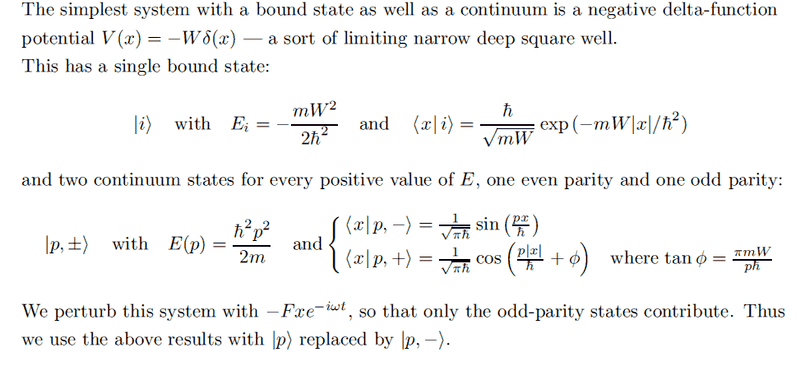# Delta Potential - Bound and Continuum States

unscientific

## Homework Statement

I am studying my lecturer's notes and in this part he uses a delta potential to illustrate a simple example of Fermi's golden rule, that the rate of excitation is ##\propto t##.## The Attempt at a Solution

I've managed to get the bound states, by solving boundary conditions. They turn out to be ##\frac{\sqrt{Wm}}{\hbar}exp(-\frac {mW|x|}{\hbar^2})##.

How are the continuum states derived? And why do only odd parity states contribute, when there is a perturbation ##-Fxe^{-i\omega t}##?

Homework Helper
Gold Member
The continuum states can be derived is a similar manner to the bound state. You can assume the solutions are either even or odd functions. Start by thinking about the general form of the solutions for E>0 for the regions x>0 and x<0.

Fermi's golden rule contains a matrix element of the perturbation function between the initial and final states. Consider the overall parity of the integrand that expresses the matrix element.

unscientific
The continuum states can be derived is a similar manner to the bound state. You can assume the solutions are either even or odd functions. Start by thinking about the general form of the solutions for E>0 for the regions x>0 and x<0.

Fermi's golden rule contains a matrix element of the perturbation function between the initial and final states. Consider the overall parity of the integrand that expresses the matrix element.

The matrix element in the integral is: ##|<p|V|i>|^2 = \left(\int \psi_p^* V \psi_i\right)^2##

If ##\psi_p## is odd, multiplied by ##V## which is odd, together they form an even function so the integral ##\int_{-\infty}^{\infty} = 2\int_0^{\infty}## which gives a non-zero value.

Homework Helper
Gold Member
That's right. So the odd ##\psi_p## will generally have a nonzero matrix element. Similarly, you can show that the even ##\psi_p## must yield zero for the matrix element.